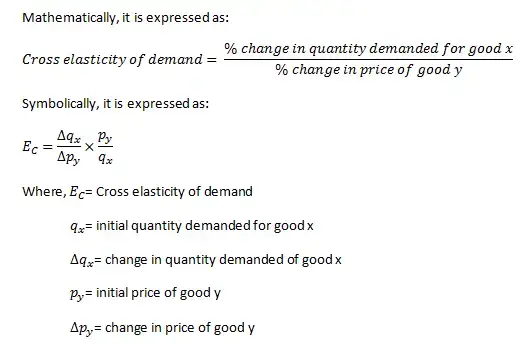# Cross Price Elasticity of Demand

The cross-price elasticity of demand is the degree of responsiveness of quantity demanded of a commodity due to the change in price of another commodity.

### Mathematical Formula for Cross Elasticity of Demand

Cross elasticity of demand is the percentage change in the quantity demanded of good X due to certain percent change in the price of good Y.### Numerical Example to Explain Cross Elasticity of Demand

Tea and coffee are substitutes to each other. If the price of coffee rises from Rs.10 per 100 grams to Rs.15 per 100 grams and as a result, consumer demand for tea increases from 30/100 grams to 40/100 grams, find out the cross elasticity of demand between tea and coffee.

Here, If we suppose tea as good x and coffee as good y.Thus, the coefficient of cross elasticity is 2/3 which shows that the quantity demanded for tea increases 2% when the price of coffee rises by 3%.

### Types of Cross Elasticity of Demand

#### Positive cross elasticity of demand (EC>0)

If rise in price of one good leads to rise in quantity demanded of other good of a similar nature and vice versa, it is known as positive cross elasticity of demand. Positive cross elasticity exists between two goods which are substitutes of each other.In the above figure, quantity demanded for Coke and price of Pepsi are measured along X-axis and Y-axis respectively. When the price of Pepsi increases from OP to OP1, quantity demanded for coke rises from OQ to OQ1 and vice versa. Thus, the demand curve DD shows positive cross elasticity of demand.

#### Negative cross elasticity of demand (EC<0)

Two goods which are complementary have negative cross elasticity of demand. If the rise in price of one good leads to fall in quantity demanded of its complementary good and vice versa, it is known as negative cross elasticity of demand.In the above figure, quantity demanded for Tea and price of Sugar are measured along X-axis and Y-axis respectively. When the price of Sugar increases from OP to OP1, quantity demanded for Tea falls from OQ to OQ1 and vice versa. Thus, the demand curve DD shows negative cross elasticity of demand.